# US8860518B1 - Current-feedback operational-amplifier based relaxation oscillator - Google Patents

## Info

Publication number
US8860518B1
US8860518B1 US14/162,339 US201414162339A US8860518B1 US 8860518 B1 US8860518 B1 US 8860518B1 US 201414162339 A US201414162339 A US 201414162339A US 8860518 B1 US8860518 B1 US 8860518B1
Authority
US
United States
Prior art keywords
sat
capacitive
terminal
current
directly coupled
Prior art date
Legal status (The legal status is an assumption and is not a legal conclusion. Google has not performed a legal analysis and makes no representation as to the accuracy of the status listed.)
Active
Application number
US14/162,339
Inventor
Zainulabideen Jamal Khalifa
Current Assignee (The listed assignees may be inaccurate. Google has not performed a legal analysis and makes no representation or warranty as to the accuracy of the list.)
King Fahd University of Petroleum and Minerals
Original Assignee
King Fahd University of Petroleum and Minerals
Priority date (The priority date is an assumption and is not a legal conclusion. Google has not performed a legal analysis and makes no representation as to the accuracy of the date listed.)
Filing date
Publication date
Application filed by King Fahd University of Petroleum and Minerals filed Critical King Fahd University of Petroleum and Minerals
Priority to US14/162,339 priority Critical patent/US8860518B1/en
Assigned to KING FAHD UNIVERSITY OF PETROLEUM AND MINERALS reassignment KING FAHD UNIVERSITY OF PETROLEUM AND MINERALS ASSIGNMENT OF ASSIGNORS INTEREST (SEE DOCUMENT FOR DETAILS). Assignors: KHALIFA, ZAINULABIDEEN JAMAL, ABUELMA'ATTI, MUHAMMAD TAHER
Application granted granted Critical
Publication of US8860518B1 publication Critical patent/US8860518B1/en
Application status is Active legal-status Critical
Anticipated expiration legal-status Critical

• 239000011651 chromium Substances 0 claims description 21
• 239000003990 capacitor Substances 0 claims description 18
• 230000001747 exhibited Effects 0 abstract 1
• 238000007600 charging Methods 0 description 4
• 238000000034 methods Methods 0 description 4
• 238000007689 inspection Methods 0 description 3
• 230000003071 parasitic Effects 0 description 3
• 238000005225 electronics Methods 0 description 2
• 238000004377 microelectronics Methods 0 description 2
• 239000000872 buffers Substances 0 description 1
• 230000036039 immunity Effects 0 description 1
• 230000001939 inductive effects Effects 0 description 1
• 230000035945 sensitivity Effects 0 description 1

## Images

•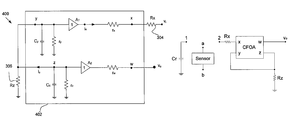•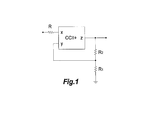•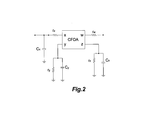•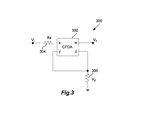•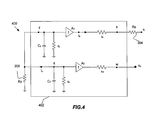•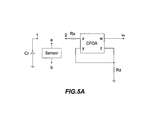•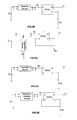•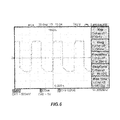•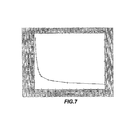•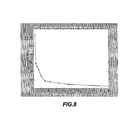## Classifications

• HELECTRICITY
• H03BASIC ELECTRONIC CIRCUITRY
• H03KPULSE TECHNIQUE
• H03K3/00Circuits for generating electric pulses; Monostable, bistable or multistable circuits
• H03K3/02Generators characterised by the type of circuit or by the means used for producing pulses
• H03K3/023Generators characterised by the type of circuit or by the means used for producing pulses by the use of differential amplifiers or comparators, with internal or external positive feedback
• H03K3/0231Astable circuits

## Abstract

A current-feedback operational-amplifier Based Relaxation oscillator provides oscillation based on a current feedback operation amplifier and two external resistors which exhibit a low output impedance terminal. This current-feedback operational-amplifier Based oscillator is used as a component for a capacitive, resistive and capacitive-resistive sensor electronic interfacing circuit.

## Description

BACKGROUND

1. Field of the Disclosure

The exemplary implementations described herein relate to a current-feedback operational-amplifier based relaxation oscillator as a versatile electronic interface for capacitive and resistive sensors.

2. Description of the Related Art

Oscillators are widely used as electronic interface for sensors. This is attributed to their simplicity and immunity to electromagnetic interference. While harmonic oscillators, widely used for capacitive and inductive sensors, have a very high sensitivity, due to their resonant nature, relaxation oscillators are simpler and less sensitive. This explains the growing interest in designing relaxation oscillations using operational amplifiers, operational transconductance amplifiers, second-generation current-conveyors and current feedback operational amplifiers (CFOAs). Of particular interest here is the relaxation oscillators built around CFOAs. This is attributed to their higher signal bandwidths, greater linearity, wider dynamic range, simple circuitry and low power consumption.

Inspection of the available current-conveyor based relaxation oscillators shows that a Schmitt trigger is required for each circuit implementation. While some implementations use current-in current-out Schmitt triggers others use the input-voltage output-voltage Schmitt trigger shown in FIG. 1 and described in Catalodo et al., (“A Schmitt trigger by means of a CCII+, International Journal of Circuit Theory and Applications, Vol. 23, 1995, pp. 161-165-incorporated herein by reference). Inspection of FIG. 1 shows that this Schmitt trigger circuit requires three resistors and does not have a low-output impedance terminal. Moreover, using this Schmitt trigger circuit a relaxation oscillator was proposed in Almashary et al., (“Current-mode triangular wave generator using CCIIs”, Microelectronics Journal, Vol. 31, 2000, pp. 239-243-incorporated herein by reference) with the resistance R replaced by the internal parasitic resistance rx of the second-generation current conveyor; as shown in FIG. 2. Thus, cascading the relaxation oscillator circuit, based on FIG. 1, for further signal processing, may require an impedance matching circuit.

Furthermore, the relaxation oscillator reported in Almashary et al., (“Current-mode triangular wave generator using CCIIs”, Microelectronics Journal, Vol. 31, 2000, pp. 239-243-incorporated herein by reference) was used as the basis for designing a current-conveyor based relaxation oscillator versatile electronic interface for capacitive and resistive sensors as described in Abuelma'atti et al., (“A current conveyor-based relaxation oscillator as a versatile electronic interface for capacitive and resistive sensors,” International Journal of Electronics, Vol. 92, 2005, pp. 473-477—incorporated herein by reference). In the interface circuit of the parasitic resistance rx is used for deciding the frequency of oscillation of the relaxation oscillator. While the resistance rx is relatively small its value is not constant. Thus, the operation of the electronic interface reported in Abuelma'atti et al., (“A current conveyor-based relaxation oscillator as a versatile electronic interface for capacitive and resistive sensors,” International Journal of Electronics, Vol. 92, 2005, pp. 473-477—incorporated herein by reference) may not be reliable.

SUMMARY

One embodiment of the disclosure includes a current-feedback operational-amplifier based serial resistive/serial capacitive/capacitive-resistive sensor electronic interfacing circuit system, including: a first output terminal; a serial resistive/serial capacitive/capacitive-resistive sensor having a first terminal and a second terminal; a reference capacitor directly coupled between the first terminal of the serial resistive/serial capacitive/capacitive-resistive sensor and a ground; a current-feedback operational-amplifier based Schmitt trigger circuit including: a first input terminal directly coupled to the second terminal of the serial resistive/serial capacitive/capacitive-resistive sensor; a second output terminal directly coupled to the first output terminal of the serial resistive/serial capacitive/capacitive-resistive sensor; a current feedback operational amplifier having a second input terminal, a third input terminal, a third output terminal and a fourth output terminal, wherein the third output terminal directly coupled to the first output terminal of the current-feedback operational-amplifier based serial resistive/serial capacitive/capacitive-resistive sensor electronic interfacing circuit system, and the fourth output terminal directly coupled to the third input terminal; a first resistor directly coupled between the first input terminal and the second input terminal, and a second resistor directly coupled between the third input terminal and a ground.

Another embodiment of the disclosure includes a current-feedback operational-amplifier based parallel capacitive sensor electronic interfacing circuit system, comprising: a first output terminal; a parallel capacitive sensor having a first terminal and a second terminal, wherein the second terminal directly coupled to a ground; a reference capacitor directly coupled between the first terminal of the parallel capacitive sensor and the ground; a current-feedback operational-amplifier based Schmitt trigger circuit including: a first input terminal directly coupled to the first terminal of the parallel capacitive sensor; a second output terminal, directly coupled to the first output terminal of the current-feedback operational-amplifier based parallel capacitive sensor electronic interfacing circuit system; a current feedback operational amplifier having a second input terminal, a third input terminal, a third output terminal and a fourth output terminal, wherein the third output terminal directly coupled to the first output terminal of the current-feedback operational-amplifier based parallel capacitive sensor electronic interfacing circuit system, and the fourth output terminal directly coupled to the third input terminal; a first resistor directly coupled between the first input terminal of the current-feedback operational-amplifier based Schmitt trigger circuit and the second input terminal, and a second resistor directly coupled between the third input terminal and a ground.

A further embodiment of the disclosure includes a current-feedback operational-amplifier based Schmitt trigger circuit including: a first input terminal; a first output terminal; a current feedback operational amplifier having a second input terminal, a third input terminal, a second output terminal and a third output terminal, wherein the second output terminal directly coupled to the first output terminal, and the third output terminal directly coupled to the third input terminal; a first resistor directly coupled between the first input terminal and the second input terminal; a second resistor directly coupled between the third input terminal and a ground.

BRIEF DESCRIPTION OF THE DRAWINGS

FIG. 1 is a schematic drawing of a CCII+ based Schmitt trigger circuit.

FIG. 2 is a schematic drawing of an AD844 current-feedback operational-amplifier.

FIG. 3 is a schematic drawing of a proposed current-feedback operational-amplifier based Schmitt Trigger.

FIG. 4 is a schematic diagram of the proposed equivalent circuit of the current-feedback operational-amplifier based Schmitt Trigger.

FIG. 5A is a schematic drawing of a proposed current-feedback operational-amplifier based capacitive/resistive sensor electronic interfacing circuit.

FIG. 5B is a schematic drawing of a proposed current-feedback operational-amplifier based serial resistive sensor electronic interfacing circuit.

FIG. 5C is a schematic drawing of a proposed current-feedback operational-amplifier based parallel capacitive sensor electronic interfacing circuit.

FIG. 5D is a schematic drawing of a proposed current-feedback operational-amplifier based serial capacitive sensor electronic interfacing circuit.

FIG. 5E is a schematic drawing of a proposed current-feedback operational-amplifier based serial capacitive-resistive sensor electronic interfacing circuit.

FIG. 6 is a plot of output waveform obtained from the FIG. 5A with RX=10.0K, RZ=1.0K and Cr=10.0 nF.

FIG. 7 is a plot of a variation of the output frequency of the circuit of FIG. 5A with the capacitance Rz at a condition that RX=1.0K and Cr=10.0 nF.

FIG. 8 is a plot of a variation of the output frequency of the circuit of FIG. 5A with the capacitance Cr at a condition that RZ=10.0K and RX=1.0K.

DETAILED DESCRIPTION OF THE PRESENT EMBODIMENTS

The present embodiment is a Current-Feedback Operational-Amplifier Based Relaxation Oscillator (CFOA)-based Schmitt trigger circuit that uses only two resistors and enjoys a low impedance output terminal. The use of the proposed Schmitt trigger circuit in designing a reliable electronic interface for capacitive, resistive or capacitive-resistive sensors is presented.

A current-feedback operational amplifier (CFOA) based relaxation oscillator 300, as shown in FIG. 3, includes a CFOA 302, an external resistor RX 304 connected between the CFOA x input and oscillator input Vi, and an external resistor RZ 306 connected between the y and z terminals of the CFOA 302 and ground. In FIG. 3, x and y are differential inputs to the CFOA 302, and w is the output pin. The equivalent circuit of this oscillator structure is shown in FIG. 4, wherein the dotted box represents a simplified equivalent circuit for the CFOA 302. In this equivalent circuit, rx and rw represent the output resistances of the unity-gain buffers A1 and A2, respectively; Cz is the internally connected compensation capacitor; Rz is the internal resistance of the gain node; and Cy and Ry represent the input impedance at terminal y of the CFOA.

In FIG. 3 the resistance RX is an externally connected resistance with RX>>rx where rX is the parasitic internal resistance at terminal x of the CFOA. The output of the Schmitt trigger of FIG. 3 will switch between its maximum, Vsat+, and minimum, Vsat−, when the input voltage, Vi, reaches the following two threshold voltages as described in equation (1) and (2):

$V th + = R Z - R X R Z ⁢ V sat + ( 1 )$

$V th - = R Z - R X R Z ⁢ V sat - ( 2 )$

Considering the circuit of FIG. 5 with nodes 1 and 2 connected and the sensor disconnected. Assume that the capacitor Cr has an initial voltage=Vth−, and is charging through the resistance RX heading towards Vsat+. The voltage across the capacitor can be described as:
V C r (t)=V sat+−(V sat+ −V th−)exp(−t/C r R X)  (3)

The capacitor will continue charging until its voltage reaches Vth+ when the output voltage of the CFOA switches to Vsat− and the process will be reversed. Using equation (3), the time T1 required to charge the capacitor from Vth− to Vth+ will be given by:

$T 1 = C r ⁢ R X ⁢ ln ⁡ ( V sat + - V th - V sat + - V th + ) ( 4 )$

When the capacitor voltage reaches Vth+ the output voltage of the CFOA switches to Vsat− and the capacitor starts a charging process heading towards Vsat−. The voltage across the capacitor can be described as:
V C r (t)=V sat−−(V sat− −V th+)exp(−t/C r R X)  (5)

The capacitor will continue charging until its voltage reaches Vth− when the output voltage of the CFOA switches to Vsat+ and the process will be reversed. Using equation (5), the time T2 required to charge the capacitor from Vth+ to Vth− will be given by:

$T 2 = C r ⁢ R X ⁢ ln ⁡ ( V sat - - V th + V sat - - V th - ) ( 6 )$

When the voltage across the capacitor reaches Vth− the output voltage of the CFOA switches to Vsat+ and the process will be repeated. Using equations (4) and (6) the duration of the resulting oscillation period will be given by

$T = T 1 + T 2 = 1 f = C r ⁢ R X ⁢ ln ⁡ ( V sat + - V th - ⁢ V sat - - V th + V sat + - V th + ⁢ V sat - - V th - ) ( 7 )$

Inspection of equations (1), (2), (4) and (6) clearly shows that a square-wave with duty cycle different from 50% can be obtained by proper selection of Vsat+ and Vsat−.

Combining equations (1), (2) and (7) yields

$T = T 1 + T 2 = 1 f = C r ⁢ R X ⁢ ln ⁡ ( ( R Z R X - R Z - R X R X ⁢ V sat - V sat + ) ⁢ ( R Z R X - R Z - R X R X ⁢ V sat + V sat - ) ) ( 8 )$

For the special case with Vsat−=Vsat+ equation (8) reduces to

$T = T 1 + T 2 = 1 f = C r ⁢ R X ⁢ ln ⁡ ( 2 ⁢ ⁢ R Z R X - 1 ) ( 9 )$

In the proposed CFOA-based capacitive/resistive sensor electronic interfacing circuit shown in FIG. 5A the dotted box can be:

For case (a), as shown in FIG. 5B, a resistive sensor with resistance Rs connected in series with the reference capacitance Cr; that is with terminal a connected to terminal 1 and terminal b connected to terminal 2. In this case the resistance RX in equations (8) and (9) will be replaced by RST=Rs+RX, and the oscillation period of serial resistive sensor network can be described as:

$T = T 1 + T 2 = 1 f = C r ⁢ R ST ⁢ ln ⁡ ( ( R Z R ST - R Z - R ST R ST ⁢ V sat - V sat + ) ⁢ ( R Z R ST - R Z - R ST R ST ⁢ V sat + V sat - ) ) ( 10 )$

For the special case with Vsat−=−Vsat+ equation (10) reduces to

$T = T 1 + T 2 = 1 f = 2 ⁢ C r ⁢ R ST ⁢ ln ⁡ ( 2 ⁢ ⁢ R Z R ST - 1 ) ( 11 )$

For case (b), as shown in FIG. 5C, a capacitive sensor with capacitance Cs connected in parallel with the reference capacitor Cr; that is with terminal a connected to terminal 1 and terminal 2 and terminal b connected to the ground. In this case the capacitance Cr in equations (8) and (9) will be replaced by CPT=Cr+Cs and the oscillation period of parallel capacitive sensor network is described as:

$T = T 1 + T 2 = 1 f = C PT ⁢ R X ⁢ ln ⁡ ( ( R Z R X - R Z - R X R X ⁢ V sat - V sat + ) ⁢ ( R Z R X - R Z - R X R X ⁢ V sat + V sat - ) ) ( 12 )$

For the special case with Vsat−=−Vsat+ equation (12) reduces to

$T = T 1 + T 2 = 1 f = 2 ⁢ C PT ⁢ R X ⁢ ln ⁡ ( 2 ⁢ ⁢ R Z R ST - 1 ) ( 13 )$

For case (c), as shown in FIG. 5D, a capacitive sensor with capacitance Cs connected in series with the reference capacitor Cr; that is with terminal a connected to terminal 1 and terminal b connected to terminal 2. In this case the capacitance Cr in equations (8) and (9) will be replaced by CST≅CrCs/(Cr+Cs) and the oscillation period of serial capacitive sensor network is described as:

$T = T 1 + T 2 = 1 f = C ST ⁢ R X ⁢ ln ⁡ ( ( R Z R X - R Z - R X R X ⁢ V sat - V sat + ) ⁢ ( R Z R X - R Z - R X R X ⁢ V sat + V sat - ) ) ( 14 )$

For the special case with Vsat−=−Vsat+ equation (14) reduces to

$T = T 1 + T 2 = 1 f = 2 ⁢ C ST ⁢ R X ⁢ ln ⁡ ( 2 ⁢ ⁢ R Z R X - 1 ) ( 15 )$

For case (d), as shown in FIG. 5E, a capacitive-resistive sensor with capacitance Cs and resistance connected Rs in series with the reference capacitor Cr; that is with terminal a connected to terminal 1 and terminal b connected to terminal 2. In this case the capacitance Cr and the resistance RX in equations (8) and (9) will be replaced by CT≅CrCs/(Cr+Cs) and RT≅RX+RS respectively, and the oscillation period of capacitive-resistive sensor network is described as:

$T = T 1 + T 2 = 1 f = C T ⁢ R X ⁢ ln ⁡ ( ( R Z R T - R Z - R T R T ⁢ V sat - V sat + ) ⁢ ( R Z R T - R Z - R T R T ⁢ V sat + V sat - ) ) ( 16 )$

For the special case with Vsat−=−Vsat+ equation (16) reduces to

$T = T 1 + T 2 = 1 f = 2 ⁢ C T ⁢ R X ⁢ ln ⁡ ( 2 ⁢ ⁢ R Z R T - 1 ) ( 17 )$

The approximations made in cases (a) and (d) are based on the assumptions that the time constant Cxrx<<CTRs.

The proposed circuit of FIG. 5A was tested using the current-feedback operational-amplifier AD844 (Spice model available from Analog Device is shown in FIG. 2 using the following values of the components: Rz≅3, MΩ, CZ≅4.5 pF, Ry≅10 MΩ, Cy≈2.0 pF, and rx≈50Ω). By selecting Cr=10 nF and Rx=10 kΩ, then RZ=1.0K, rectangular oscillations are obtained, as shown in plot of FIG. 6. The variations of the frequency of oscillation with the capacitance Cr and the resistance RZ are shown in FIGS. 7 and 8. These results are obtained with DC supply voltage=±10V.

## Claims (7)

The invention claimed is:
1. A current-feedback operational-amplifier based serial resistive/serial capacitive/capacitive—resistive sensor electronic interfacing circuit system, comprising:
a first output terminal;
a serial resistive/serial capacitive/capacitive-resistive sensor having a first terminal and a second terminal;
a reference capacitor directly coupled between the first terminal of the serial resistive/serial capacitive/capacitive-resistive sensor and a ground;
a current-feedback operational-amplifier based Schmitt trigger circuit including:
a first input terminal directly coupled to the second terminal of the serial resistive/serial capacitive/capacitive-resistive sensor;
a second output terminal directly coupled to the first output terminal of the serial resistive/serial capacitive/capacitive-resistive sensor;
a current feedback operational amplifier having a second input terminal, a third input terminal, a third output terminal and a fourth output terminal, wherein the third output terminal directly coupled to the first output terminal of the current-feedback operational-amplifier based serial resistive/serial capacitive/capacitive-resistive sensor electronic interfacing circuit system, and the fourth output terminal directly coupled to the third input terminal;
a first resistor directly coupled between the first input terminal and the second input terminal, and
a second resistor directly coupled between the third input terminal and a ground.
2. The current-feedback operational-amplifier based serial resistive sensor electronic interfacing circuit system of claim 1, wherein a oscillation period of the serial resistive sensor electronic interfacing circuit system is calculated according to:
$T = C r ⁢ R ST ⁢ ln ⁡ ( ( R Z R ST - R Z - R ST R ST ⁢ V sat - V sat + ) ⁢ ( R Z R ST - R Z - R ST R ST ⁢ V sat + V sat - ) )$
where Vsat+ is a maximum output voltage of the Schmitt trigger circuit, Vsat− is a minimum output voltage of the Schmitt trigger circuit, RX is a first resistance of the first resistor, RZ is a second resistance of the second resistor, Rs is a third resistance of the serial resistivity sensor, Cr is a first capacitance of the reference capacitance and RST=Rs+RX.
3. The current-feedback operational-amplifier based serial capacitive electronic interfacing circuit system of claim 1, wherein a oscillation period of the serial capacitive sensor electronic interfacing circuit system is calculated according to:
$T = C ST ⁢ R X ⁢ ln ⁡ ( ( R Z R X - R Z - R X R X ⁢ V sat - V sat + ) ⁢ ( R Z R X - R Z - R X R X ⁢ V sat + V sat - ) )$
where Vsat+ is a maximum output voltage of the Schmitt trigger circuit, Vsat− is a minimum output voltage of the Schmitt trigger circuit, RX is a first resistance of the first resistor, RZ is a second resistance of the second resistor, Cr is a first capacitance of the reference capacitance, Cs is a second capacitance of the serial capacitive sensor, and CST≅CrCs/(Cr+Cs).
4. The current-feedback operational-amplifier based serial capacitive-resistive sensor electronic interfacing circuit system of claim 1, wherein a oscillation period of the capacitive-resistive sensor electronic interfacing circuit system network is calculated according to:
$T = C T ⁢ R X ⁢ ln ⁡ ( ( R Z R T - R Z - R T R T ⁢ V sat - V sat + ) ⁢ ( R Z R T - R Z - R T R T ⁢ V sat + V sat - ) )$
where Vsat+ is a maximum output voltage of the Schmitt trigger circuit, Vsat− is a minimum output voltage of the Schmitt trigger circuit, RX is a first resistance of the first resistor, RZ is a second resistance of the second resistor, Rs is a third resistance of the capacitive-resistive sensor, Cr is a first capacitance of the reference capacitance, Cs is a second capacitance of the capacitive-resistive sensor, CT≅CrCs/(Cr+Cs) and RT≅RX+Rs.
5. A current-feedback operational-amplifier based parallel capacitive sensor electronic interfacing circuit system, comprising:
a first output terminal;
a parallel capacitive sensor having a first terminal and a second terminal, wherein the second terminal directly coupled to a ground;
a reference capacitor directly coupled between the first terminal of the parallel capacitive sensor and the ground;
a current-feedback operational-amplifier based Schmitt trigger circuit including:
a first input terminal directly coupled to the first terminal of the parallel capacitive sensor;
a second output terminal, directly coupled to the first output terminal of the current-feedback operational-amplifier based parallel capacitive sensor electronic interfacing circuit system;
a current feedback operational amplifier having a second input terminal, a third input terminal, a third output terminal and a fourth output terminal, wherein the third output terminal directly coupled to the first output terminal of the current-feedback operational-amplifier based parallel capacitive sensor electronic interfacing circuit system, and the fourth output terminal directly coupled to the third input terminal;
a first resistor directly coupled between the first input terminal of the current-feedback operational-amplifier based Schmitt trigger circuit and the second input terminal, and
a second resistor directly coupled between the third input terminal and a ground.
6. The current-feedback operational-amplifier based parallel capacitive sensor electronic interfacing circuit system of claim 5, wherein a oscillation period of the parallel capacitive sensor electronic interfacing circuit system is calculated according to:
$T = C PT ⁢ R X ⁢ ln ⁡ ( ( R Z R X - R Z - R X R X ⁢ V sat - V sat + ) ⁢ ( R Z R X - R Z - R X R X ⁢ V sat + V sat - ) )$
where Vsat+ is a maximum output voltage of the Schmitt trigger circuit, Vsat− is a minimum output voltage of the Schmitt trigger circuit, RX is a first resistance of the first resistor, RZ is a second resistance of the second resistor, Cr is a first capacitance of the reference capacitance, Cs is a second capacitance of the parallel capacitive sensor and CPT=Cr+Cs.
7. A current-feedback operational-amplifier based Schmitt trigger circuit comprising:
a first input terminal;
a first output terminal;
a current feedback operational amplifier having a second input terminal, a third input terminal, a second output terminal and a third output terminal, wherein the second output terminal directly coupled to the first output terminal, and the third output terminal;
directly coupled to the third input terminal;
a first resistor directly coupled between the first input terminal and the second input terminal;
a second resistor directly coupled between the third input terminal and a ground.
US14/162,339 2014-01-23 2014-01-23 Current-feedback operational-amplifier based relaxation oscillator Active US8860518B1 (en)

## Priority Applications (1)

Application Number Priority Date Filing Date Title
US14/162,339 US8860518B1 (en) 2014-01-23 2014-01-23 Current-feedback operational-amplifier based relaxation oscillator

## Applications Claiming Priority (1)

Application Number Priority Date Filing Date Title
US14/162,339 US8860518B1 (en) 2014-01-23 2014-01-23 Current-feedback operational-amplifier based relaxation oscillator

## Publications (1)

Publication Number Publication Date
US8860518B1 true US8860518B1 (en) 2014-10-14

# Family

## Family Applications (1)

Application Number Title Priority Date Filing Date
US14/162,339 Active US8860518B1 (en) 2014-01-23 2014-01-23 Current-feedback operational-amplifier based relaxation oscillator

## Country Status (1)

US (1) US8860518B1 (en)

## Cited By (4)

* Cited by examiner, † Cited by third party
Publication number Priority date Publication date Assignee Title
US9019030B1 (en) * 2014-09-18 2015-04-28 King Fahd University Of Petroleum And Minerals Memristor-based emulator for use in digital modulation
US9184734B1 (en) * 2014-11-04 2015-11-10 King Fahd University Of Petroleum And Minerals Voltage or current controlled current-feedback operational-amplifier based multivibrator
US9503098B1 (en) 2016-04-06 2016-11-22 King Fahd University Of Petroleum And Minerals Current-feedback operational amplifier-based sinusoidal oscillator
US9619596B2 (en) * 2015-06-23 2017-04-11 King Fahd University Of Petroleum And Minerals Floating memristor emulator

## Citations (3)

* Cited by examiner, † Cited by third party
Publication number Priority date Publication date Assignee Title
US4115748A (en) * 1976-03-18 1978-09-19 Tokyo Shibaura Electric Co., Ltd. MOS IC Oscillation circuit
US5438292A (en) * 1992-04-07 1995-08-01 Oki Electric Industry, Co., Ltd. Schmitt trigger circuit with CMOS inverters and filtering means
US8188773B1 (en) * 2011-03-29 2012-05-29 King Fahd University Of Petroleum & Minerals Voltage-controlled dual-slope square and triangular waveform generator

## Patent Citations (3)

* Cited by examiner, † Cited by third party
Publication number Priority date Publication date Assignee Title
US4115748A (en) * 1976-03-18 1978-09-19 Tokyo Shibaura Electric Co., Ltd. MOS IC Oscillation circuit
US5438292A (en) * 1992-04-07 1995-08-01 Oki Electric Industry, Co., Ltd. Schmitt trigger circuit with CMOS inverters and filtering means
US8188773B1 (en) * 2011-03-29 2012-05-29 King Fahd University Of Petroleum & Minerals Voltage-controlled dual-slope square and triangular waveform generator

## Non-Patent Citations (2)

* Cited by examiner, † Cited by third party
Title
Martin Janecek, et al., "Voltage-Controlled Square/Triangular Wave Generator with Current Conveyors and Switching Diodes", Dec. 2012, (4 pages).
Shahram Minaei, et al., "A Simple Schmitt Trigger Circuit with Grounded passive Elements and It's Application to Square/Triangular Wave Generator", Jun. 2012, Circuts, Systems, and Signal Processing, vol. 31, Issue 3 (4 pages).

## Cited By (4)

* Cited by examiner, † Cited by third party
Publication number Priority date Publication date Assignee Title
US9019030B1 (en) * 2014-09-18 2015-04-28 King Fahd University Of Petroleum And Minerals Memristor-based emulator for use in digital modulation
US9184734B1 (en) * 2014-11-04 2015-11-10 King Fahd University Of Petroleum And Minerals Voltage or current controlled current-feedback operational-amplifier based multivibrator
US9619596B2 (en) * 2015-06-23 2017-04-11 King Fahd University Of Petroleum And Minerals Floating memristor emulator
US9503098B1 (en) 2016-04-06 2016-11-22 King Fahd University Of Petroleum And Minerals Current-feedback operational amplifier-based sinusoidal oscillator

## Similar Documents

Publication Publication Date Title
Prescott Loss-compensated active gyrator using differential-input operational amplifiers
US6194903B1 (en) Circuit for acquisition of the capacitance or capacitance change of a capacitive circuit element or component
Prokop et al. New modern circuit block CCTA and some its applications
Cakir et al. Novel allpass filter configuration employing single OTRA
Lahiri Novel voltage/current-mode quadrature oscillator using current differencing transconductance amplifier
Veeravalli et al. A CMOS transconductance amplifier architecture with wide tuning range for very low frequency applications
US3435375A (en) Controller having fet bridge circuit
Tangsrirat et al. Resistorless realization of current-mode first-order allpass filter using current differencing transconductance amplifiers
Lo et al. Switch-controllable OTRA-based square/triangular waveform generator
Munoz-Pacheco et al. Frequency limitations in generating multi-scroll chaotic attractors using CFOAs
Yuce On the realization of the floating simulators using only grounded passive components
US20010008478A1 (en) Linear capacitance measurement circuit
Almashary et al. Current-mode triangular wave generator using CCIIs
JPWO2010016167A1 (en) Reference frequency generation circuit, semiconductor integrated circuit, electronic equipment
Keskin* Voltage-mode high-Q band-pass filters and oscillators employing single CDBA and minimum number of components
Sotner et al. Current gain controlled CCTA and its application in quadrature oscillator and direct frequency modulator
Gupta et al. OTRA-based grounded-FDNR and grounded-inductance simulators and their applications
Sotner et al. Z-copy controlled-gain voltage differencing current conveyor: advanced possibilities in direct electronic control of first-order filter
JP2005301974A (en) Coordinate position detector
US20040201419A1 (en) Amplifying circuit
CN102968224B (en) The control circuit of contact panel and control method
CN100447822C (en) Human body detection sensor
US8604809B2 (en) Current sensor capacity measuring system
Lahiri et al. Voltage-mode quadrature sinusoidal oscillator with current tunable properties
Prasad et al. Realisation of single-resistance-controlled sinusoidal oscillator: a new application of the CDTA

## Legal Events

Date Code Title Description
AS Assignment

Owner name: KING FAHD UNIVERSITY OF PETROLEUM AND MINERALS, SA

Free format text: ASSIGNMENT OF ASSIGNORS INTEREST;ASSIGNORS:ABUELMA'ATTI, MUHAMMAD TAHER;KHALIFA, ZAINULABIDEEN JAMAL;SIGNING DATES FROM 20140106 TO 20140116;REEL/FRAME:032036/0815

STCF Information on status: patent grant

Free format text: PATENTED CASE

MAFP Maintenance fee payment

Free format text: PAYMENT OF MAINTENANCE FEE, 4TH YR, SMALL ENTITY (ORIGINAL EVENT CODE: M2551)

Year of fee payment: 4